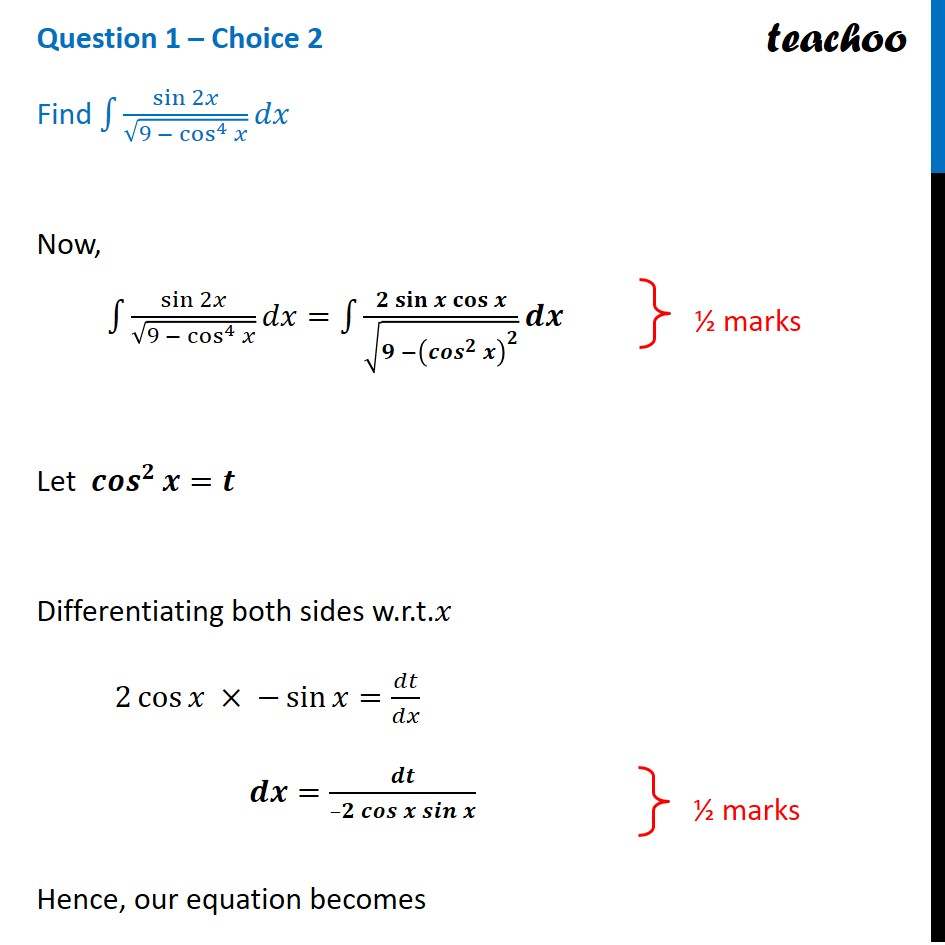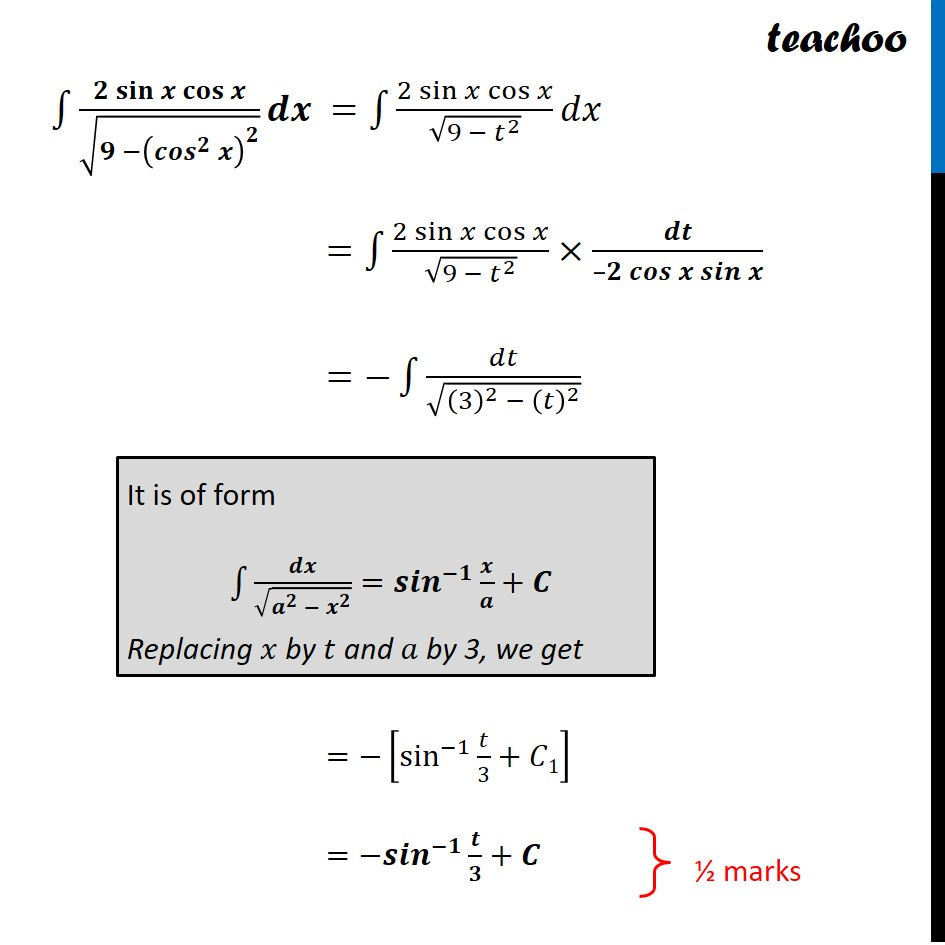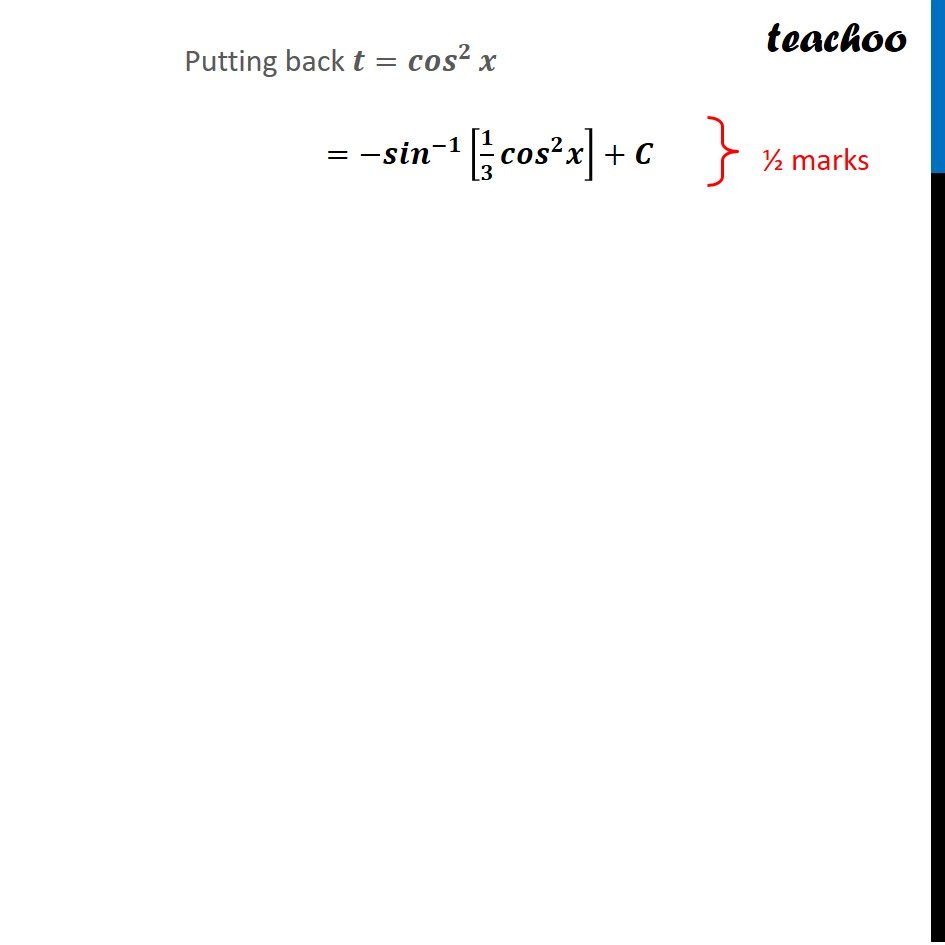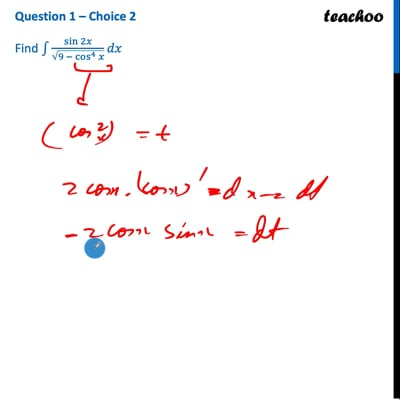CBSE Class 12 Sample Paper for 2022 Boards (For Term 2)

Class 12
Solutions of Sample Papers and Past Year Papers - for Class 12 Boards

## Find f sin 2 x/ 9 - cos4 x dx

This question is similar to Example 42 - Chapter 7 Class 12 - IntegralsThis video is only available for Teachoo black users

Introducing your new favourite teacher - Teachoo Black, at only ₹83 per month

### Transcript

Question 1 – Choice 2 Find ∫1▒〖sin⁡2𝑥/√(9 − cos^4⁡𝑥 ) 𝑑𝑥〗Now, ∫1▒〖sin⁡2𝑥/√(9 − cos^4⁡𝑥 ) 𝑑𝑥〗=∫1▒〖(𝟐 𝐬𝐢𝐧⁡𝒙 𝐜𝐨𝐬⁡𝒙)/√(𝟗 −(〖𝒄𝒐𝒔〗^𝟐⁡𝒙 )^𝟐 ) 𝒅𝒙〗 Let 〖𝒄𝒐𝒔〗^𝟐⁡𝒙=𝒕 Differentiating both sides w.r.t.𝑥 2 cos⁡𝑥 × −sin⁡𝑥=𝑑𝑡/𝑑𝑥 𝒅𝒙=𝒅𝒕/(–𝟐 𝒄𝒐𝒔⁡𝒙 𝒔𝒊𝒏⁡𝒙 ) Hence, our equation becomes ∫1▒〖(𝟐 𝐬𝐢𝐧⁡𝒙 𝐜𝐨𝐬⁡𝒙)/√(𝟗 −(〖𝒄𝒐𝒔〗^𝟐⁡𝒙 )^𝟐 ) 𝒅𝒙〗 =∫1▒〖(2 sin⁡𝑥 cos⁡𝑥)/√(9 − 𝑡^2 ) 𝑑𝑥〗 =∫1▒〖(2 sin⁡𝑥 cos⁡𝑥)/√(9 − 𝑡^2 )×𝒅𝒕/(–𝟐 𝒄𝒐𝒔⁡𝒙 𝒔𝒊𝒏⁡𝒙 )〗 =−∫1▒𝑑𝑡/√((3)^2 − (𝑡)^2 ) =−[sin^(−1)⁡〖𝑡/3+𝐶1〗 ] =−𝒔𝒊𝒏^(−𝟏) 𝒕/𝟑+𝑪 It is of form ∫1▒〖𝒅𝒙/√(𝒂^𝟐 − 𝒙^𝟐 )=𝒔𝒊𝒏^(−𝟏) 𝒙/𝒂+𝑪〗 Replacing 𝑥 by 𝑡 and 𝑎 by 3, we get Putting back 𝒕=〖𝒄𝒐𝒔〗^𝟐⁡𝒙 =−𝒔𝒊𝒏^(−𝟏) [𝟏/𝟑 𝒄𝒐𝒔^𝟐 𝒙]+𝑪